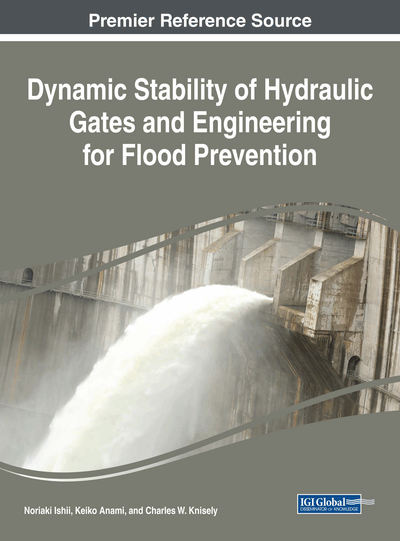# Streamwise Gate Vibration: Added Mass and Damping

DOI: 10.4018/978-1-5225-3079-4.ch005

## Abstract

Streamwise vibrations of gates due to the bending flexibility of the skinplate of Tainter gates or the weir plate of long-span gates result in pushing-and-drawing of the water in the reservoir. During each cycle of vibration, the gate's motion must accelerate and then decelerate the water mass in contact with the vibrating gate surface, resulting in a substantial added mass effect. From simple single degree-of-freedom mass-spring-damper vibration theory, one understands that the effect of added mass is to lower the frequency of gate vibration. In addition to the push-and-draw effect, streamwise motion can also result in discharge fluctuation for inclined gates, providing a source of gate excitation. Rayleigh's wave theory analysis from the previous chapter is applied to provide an analysis framework for determining the magnitude of wave radiation damping and to calculate the added mass.
Chapter Preview
Top

## Theoretical Analysis Of Push-And-Draw Pressure

In Chapter 4, Rayleigh’s wave theory was used to analyze the effect of small amplitude vertical vibrations of a vertical weir plate on the flow field. In this chapter, the same framework will be used to consider the hydrodynamic effects of small amplitude horizontal vibrations of the vertical weir plate.

The simplifications of the bounding geometry and boundary conditions that we will use for the present theoretical analysis of streamwise weir plate vibration are similar to those in the previous chapter. Figure 1 shows the simplified geometry in which the curved skinplate and the dam wall of the Tainter gate (see Figure 1 in Chapter 4) are replaced with a vertical planar weir plate oriented parallel to the Y-axis, while the inclined weir plate of the long-span gate (see Figure 2 in Chapter 4) is assumed to stand vertically along the Y-axis. The still water surface is used to define the X-axis. The analysis is done for a finite depth reservoir, which can then be extended to infinite depth reservoirs as well.

## Complete Chapter List

Search this Book:
Reset